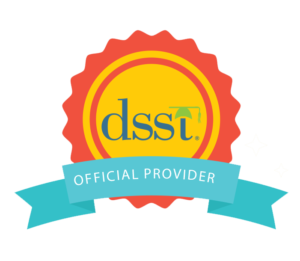If you excel in calculus, linear algebra, data analysis and the theory of statistics and are looking to earn college credit, the DSST® Principles of Statistics exam is just for you. DSST exams help you earn college credit while saving you time and money, with the average college course costing over \$900.

Let’s take a deep dive into what’s included in the DSST Principles of Statistics exam and how to prepare for it.

### Inside the DSST Principles of Statistics exam

The DSST Principles of Statistics exam, which consists of 100 multiple-choice questions, covers topics such as probability, correlation, regression, sampling distribution, and inferential statistics.

Credit amount: 3 semester hours

Minimum passing score: 400

The topics covered in the exam and the weight of each topic include:

1. Foundations of statistics – 20%
2. Probability – 20%
3. Correlation and Regression – 15%
4. Sampling Distributions – 15%
5. Inferential Statistics – 30%

### Sample questions

1. A farmer wants to test 4 different fertilizers to see which one produces the tallest tomato plants. He divides his field into 4 sections, and uses a different fertilizer in each. At the end of the growing season, he measures the heights of a sample of tomato plants from each section, and finds the means of each sample. What type of hypothesis test would be most appropriate for him to conduct?
A. ANOVA
B. t-test for a mean
C. t-test for the difference of two means
D. z-test for a proportion
2. If samples of a sufficiently large size are repeatedly taken from a large population, which of the following is NOT necessarily true about the distribution of sample means?
A. It is symmetric.
B. It can be well approximated by a normal distribution.
C. The mean is the same as the population mean.
D. The standard deviation is the same as the population standard deviation.
3. Which of the following descriptive statistics are influenced by outliers?
A. Mean and median
B. Mean and standard deviation
C. Median and IQR
D. Standard deviation and IQR

### Preparing for the examPeterson’s DSST test prep encompasses 37 DSST exams, and includes 3 full-length practice tests, and most courses also include videos, and flashcards. Peterson’s is an official test-prep provider of the DSST, so you can be confident that what you see on our practice tests will be the types of questions to expect on exam day.